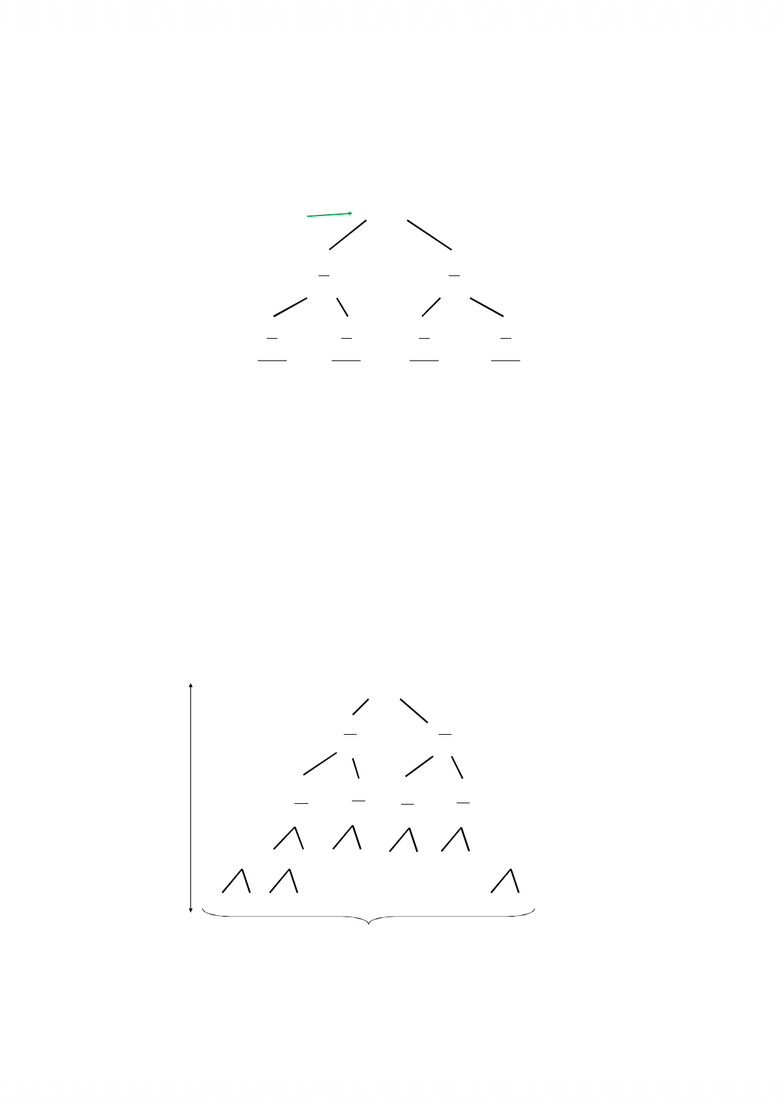# CS341 Lecture Notes - Merge Sort, Binary Search Algorithm, Dont

33 views14 pages
School
University of Waterloo
Department
Computer Science
Course
CS3411
Solving Recurrences
Method 1: Recursion Trees
Method 2: Master theorem
Prove a general theorem covering a wide variety
of recurrences.
Apply the theorem in “cookbook” fashion.
Method 3: Guessing and Checking method
Prove it using induction.
Recursion Trees: Merge Sort (page 1)
Merge sort: an analysis:
// Sort sequence A[l..r]
function merge_sort(l, r)
// Base case: 1 element is always sorted
if (l = r) then return;
m := (l + r)/2;
// We need to sort both subsequences l..m, m+1..r
merge_sort(l, m);
merge_sort(m + 1, r);
// Finally: merge the two sorted sequences
merge(l, m, r);
Unlock document

This preview shows pages 1-3 of the document.
Unlock all 14 pages and 3 million more documents.2
Recursion Trees: Merge Sort (page 2)
In the previous code we used a merge function:
// Merge two sorted sequences l..m, m+1..r
function merge(l, m, r)
copy A[l..m] to L; L[m – l + 2] := infinity;
copy A[m + 1..r] to R; R[r – m + 1] := infinity;
i := 1; j := 1; k := l;
while (L[i] < infinity or R[j] < infinity) do
if L[i] <= R[j] then
A[k] := L[i];
i := i + 1; k := k + 1;
else
A[k] := R[j];
j := j + 1; k := k + 1;
Recursion Trees: Merge Sort (page 3)
Given the recurrence for T(n):
we display the computation in the form of a tree
(one node for each time the function is invoked).
Label each node in the tree with the combine time
cost incurred at that node.
Leaves are labeled with base case cost T(0).
The sum of all costs (including the leaves) is the
total running time of the algorithm, so we sum up
all costs in a convenient way (usually level by level)
0 if 1
( )
( ) if 1
2 2
n
T n n n
T T n n
=
=   
   
+ + Θ >
   
   
   
   
Unlock document

This preview shows pages 1-3 of the document.
Unlock all 14 pages and 3 million more documents.3
Recursion Trees: Merge Sort (page 4)
This is getting messy, so we use a sloppy form of the recurrence:
an
2
n
a
 
 
 
2
n
a
 
 
 
2
2
n
a
 
 
 
NO
2
2
n
a
 
 
 
 
 
 
 
 
 
2
2
n
a
 
 
 
 
 
 
 
 
 
2
2
n
a
 
 
 
 
 
 
 
 
 
(
)
from / 2
T n
 
 
(
)
from / 2
T n
 
 
(
)
from
T n
Recursion Trees: Merge Sort (page 5)
an
a
n
2
a
n
2
an
4
an
4
an
4
1
+
log
n
levels
an
4
b b b b
b b
2
log n
=
n
an
an
an
sum: an (log n)
Work done at each level:
Unlock document

This preview shows pages 1-3 of the document.
Unlock all 14 pages and 3 million more documents.